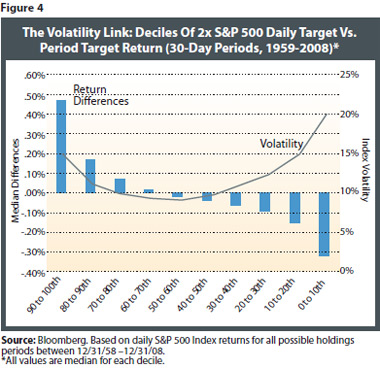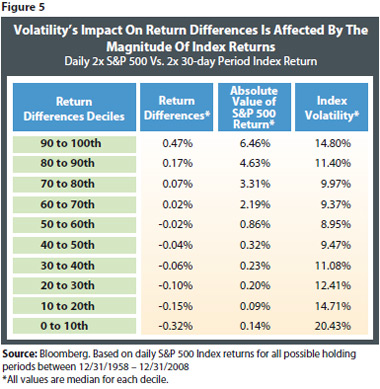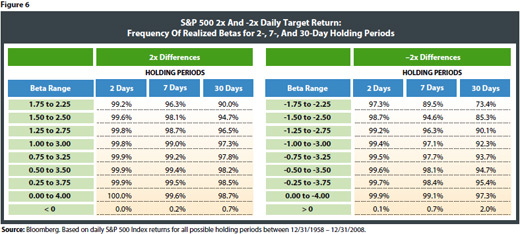# Understanding Returns Of Leveraged And Inverse Funds

August 25, 2009

Analysis Of Differences Between 2x And -2x S&P 500 Funds And Period Target Leveraged/Index Returns

Figure 3 contains statistics from the distribution of differences in return between the daily target return and the period target return (2x and -2x) for all possible two-, seven- and 30-day holding periods over the 50-year S&P 500 return history. The averages of the percentage return differences are all essentially zero, and the median is at or just below zero, indicating that leveraged and inverse strategies are about as likely to benefit as to be hurt by the compounding effect of periods up to 30 days for the S&P 500.

The distribution of the return differences for the S&P 500 2x and -2x strategies is tight and balanced over this long history. For example, half of the differences for the 2x fund for a 30-day holding period were between 0.1 percent and -0.1 percent. This means that for a hypothetical 2x fund over a 30-day period where the S&P 500 return was 3 percent, the returns were in a range of 5.9 percent to 6.1 percent (compared with 2 x 3 percent or 6 percent) approximately half the time. In addition, the percentage of positive differences was 53 percent for two-day holding periods. As we move out to longer periods, the return differences are positive about 40 percent of the time.

Volatility Drives The Differences Between Leveraged Daily Target Fund And Index Returns

What drives the size of differences between daily target leveraged and inverse returns and the period target return over a longer holding period? As noted above, we would expect the largest driver of compounding effects to be the level of volatility in the market over the investor’s holding period. This point is frequently mentioned in academic, analyst and media articles when discussing the performance differences for leveraged and inverse funds held beyond one day. Our long-term study using hypothetical 2x S&P 500 Index returns supports the view that volatility is the key factor driving the size of the differences.

To explore the connection between volatility and variability of returns over the 50-year S&P 500 return history, we first sort the return differences between the 30-day 2x fund and the S&P 500 return times two. We then place these return differences into 10 deciles, or buckets, ranking them from the most positive to the most negative. For each of these deciles, we calculate the median return difference and the median annualized 30-day S&P 500 volatility.Figure 4 displays levels of volatility that are clearly related to the magnitude of the return difference. The holding periods with the most positive and negative deciles of return differences were also ones that have higher volatility. The U shape of the median volatilities across the return difference deciles reveals that the smallest return differences tend to occur when volatility is lowest. It is notable that the recent episode of extreme volatility was the main factor in observing wider return spreads for longer holding periods for the leveraged and inverse funds.

The Impact Of Volatility Is Relative To The Magnitude Of Index Returns

The degree of impact that volatility has on leveraged fund returns is relative to the magnitude of the index return for the period. In periods when index return magnitudes are very large, the return differences tend to be large but positive. In contrast, the periods of the most negative differences (0-10th decile) are those where there have been high S&P 500 volatility levels accompanied by index returns close to zero (that is, flat or trendless markets). In Figure 5, data from our study of hypothetical 2x fund returns show the medians for each decile of return differences, along with the median volatility and absolute value of index return for each bucket. Volatility is not always unwelcome to investors pursuing returns, as higher-magnitude index returns (both positive and negative) are somewhat correlated with higher-volatility market environments.Realized Beta (Leverage) Ratios For Hypothetical 2x And -2x S&P 500 Leveraged Funds Were Close To The Daily Target Ratio